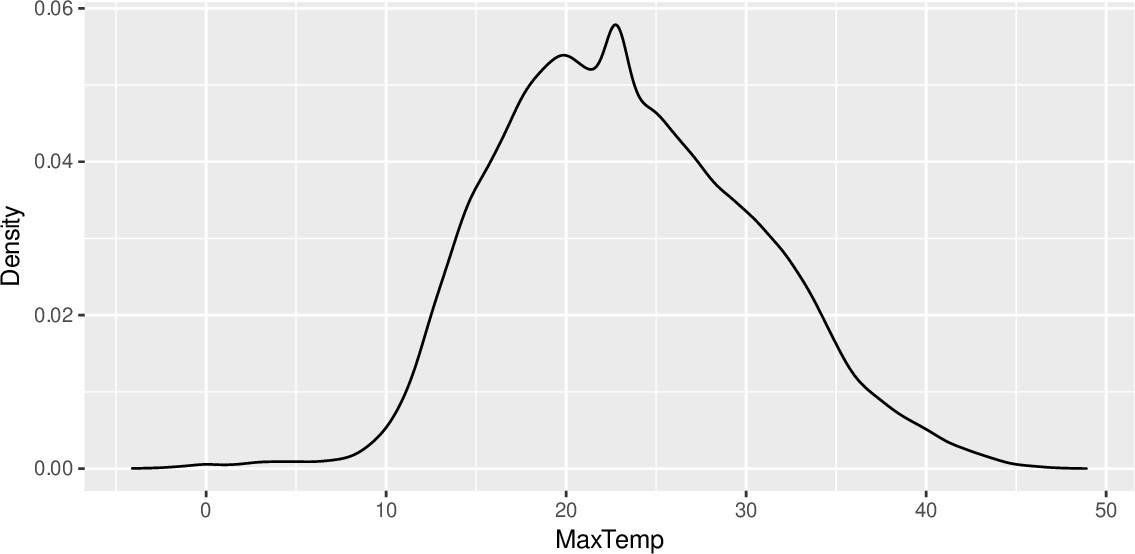Data Science Desktop Survival Guide by Graham WilliamsDesktop Survival Project Home Preface Data Science Introducing R R Constructs R Tasks R Strings R Read, Write, and Create Data Template Data Exploration Data Wrangling Data Visualisation Statistics ML Template ML Scenarios ML Activities ML Applications ML Algorithms Cluster Analysis Decision Trees Computer Vision Graph Data Privacy Literate Data Science Coding with Style Resources Bibliography Index

## Line Chart Density Distribution

20200428ds %>%   ggplot(aes(x=max_temp)) +   geom_density() +   labs(x=vnames["max_temp"], y="Density") A density plot using ggplot2::geom_density() will convey the distribution of the values of a variable. Here, for example, we can see that max_temp has a roughly normal distribution with a peak around the low 20's (degrees Celsius) and fairly short tails.